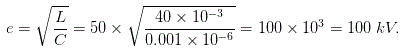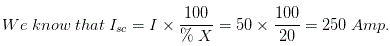# MCQs on Power Systems

##### Page 43 of 67. Go to page 1 2 3 4 5 6 7 8 9 10 11 12 13 14 15 16 17 18 19 20 21 22 23 24 25 26 27 28 29 30 31 32 33 34 35 36 37 38 39 40 41 42 43 44 45 46 47 48 49 50 51 52 53 54 55 56 57 58 59 60 61 62 63 64 65 66 67
01․ Most commonly used material for insulator is
glass
mica
porcelain
all of the above

The most commonly used material for insulators of overhead line is porcelain but glass, steatite and special composition materials are also used to a limited extent. Porcelain is produced by firing at a high temperature a mixture of kaolin, feldspar and quartz. It is stronger mechanically than glass, gives less trouble from leakage and is less affected by changes of temperature.

02․ If the breakdown voltage of the insulator is high, the thickness of the insulator is
high
less
it does not related to breakdown voltage
none of the above

The dielectric strength of a material is expressed as Volts/meter and gives the maximum electric field strength that it can withstand intrinsically without breaking down. Therefore, the thicker the layer of insulator, the higher is the breakdown voltage. The dielectric constant varies slightly with increase in thickness and temperature, but is generally assumed to be constant for simplicity.

03․ Operating time of pumped storage plant is
very high
high
low
can't say

Advantages of pumped storage plants: 1. Most economical as a peak load supply plant 2. Used as load frequency control 3. If it is combined with thermal plant of an area load factor is increased 4. Pollution free 5. Easily adaptable for remote operation 6. Operating time is minimum

04․ Advantages of per unit system is/are
No units for any quantity
Need of transformation ratio
It has units for all quantities
all of the above

Per unit of any quantity is defined as the ration of actual value of quantity to the base value of quantity in same units. Per unit of any quantity = Actual value of quantity /Base value of quantity in same units Advantages of per unit system: 1. Only one equation is required 2. Calculation time is less 3. No units for any quantity 4. No need of transformation ratio 5. Memory required is less

05․ Sparse matrix is matrix which contain
more number of non zeros
more number of memory elements
less number of memory elements
more number of zeros

A matrix is said to be a sparse matrix, when it contains more number of zero elements. Y bus matrix is a sparse matrix, containing more number of zero elements. So that faster calculation is possible.

06․ How to find the total number of strands (N) when number of layers (x) are given
Total number of strands N = 3x² + 3x + 1
Total number of strands N = 3x² - 3x - 1
Total number of strands N = 3x² + 3x - 1
Total number of strands N = 3x² - 3x + 1

Total number of strands (N) when number of layers (x) are given is Total number of strands N = 3x² - 3x + 1 Where, x = Number of layers

07․ Reactance relays are used for the protection of
short transmission lines only
long transmission lines only
medium transmission lines only
all of the above

1. Reactance relays ----- Short transmission lines 2. Impedance relays ------ Medium transmission lines 3. Mho relays ------------- Long transmission lines In short transmission line LG faults are more frequent which produces arcing grounds. This will causes the impedance relay operating point shifts towards the blocking region. So that relay may not be operated for the faults within the arcing ground. To overcome this problem reactance relay is used, it is independent of arcing ground and it is used for protection of the short transmission lines.

08․ The main objectives of power system is/are
Cost of electrical energy per KWh is to be minimum
Reliable power has to be available
Flexible power has to be available.
all of the above

Objectives of power system are 1. Cost of electrical energy per KWh is to be minimum 2. Rated voltage and frequency has to be supplied to the consumers 3. Reliable power has to be available 4. Effective protection system has to be used for isolating the faulty section and keeping other sections healthy. 5. More stable generators are to be used, so that it should not lose synchronism under faulty condition. 6. Flexible power has to be available.

09․ If L and C are 4 mH and 0.0001 ÃŽÂ¼F respectively a current chop of magnitude 50 Amp would induced a voltage
200 kV.
100 kV.
50 kV.
400 kV.10․ If the percentage reactance of an element is 20 % and the full load current is 50 Amp, the short circuit current will be
250 Amp.
300 Amp.
200 Amp.
350 Amp.<<<4142434445>>>